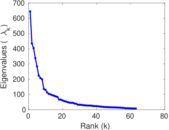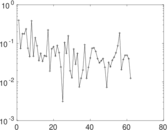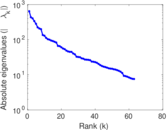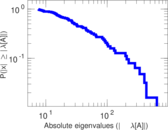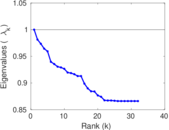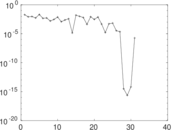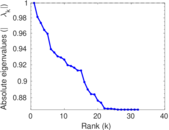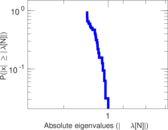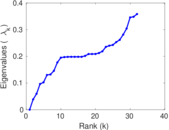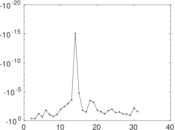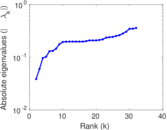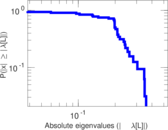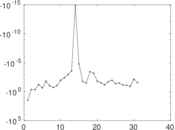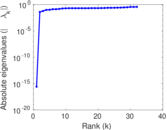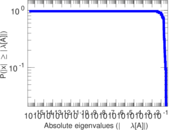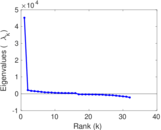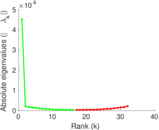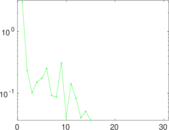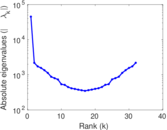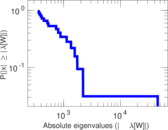# Wiktionary edits (oc)

This is the bipartite edit network of the Occitan Wiktionary. It contains users and pages from the Occitan Wiktionary, connected by edit events. Each edge represents an edit. The dataset includes the timestamp of each edit.

 Code `moc` Internal name `edit-ocwiktionary` Name Wiktionary edits (oc) Data source http://dumps.wikimedia.org/ AvailabilityDataset is available for download Consistency checkDataset passed all tests Category Authorship network Dataset timestamp 2017-10-20 Node meaning User, article Edge meaning Edit Network formatBipartite, undirected Edge typeUnweighted, multiple edges Temporal dataEdges are annotated with timestamps

## Statistics

 Size n = 45,224 Left size n1 = 426 Right size n2 = 44,798 Volume m = 275,469 Unique edge count m̿ = 148,653 Wedge count s = 797,546,209 Claw count z = 3,828,180,204,354 Cross count x = 15,436,567,238,503,784 Square count q = 660,652,251 4-Tour count T4 = 8,475,705,582 Maximum degree dmax = 38,548 Maximum left degree d1max = 38,548 Maximum right degree d2max = 632 Average degree d = 12.182 4 Average left degree d1 = 646.641 Average right degree d2 = 6.149 14 Fill p = 0.007 789 43 Average edge multiplicity m̃ = 1.853 10 Size of LCC N = 44,974 Diameter δ = 12 50-Percentile effective diameter δ0.5 = 3.179 47 90-Percentile effective diameter δ0.9 = 3.846 40 Median distance δM = 4 Mean distance δm = 3.246 32 Gini coefficient G = 0.784 124 Balanced inequality ratio P = 0.197 354 Left balanced inequality ratio P1 = 0.041 122 6 Right balanced inequality ratio P2 = 0.275 585 Relative edge distribution entropy Her = 0.688 187 Power law exponent γ = 2.182 58 Tail power law exponent γt = 4.431 00 Tail power law exponent with p γ3 = 4.431 00 p-value p = 0.000 00 Left tail power law exponent with p γ3,1 = 1.421 00 Left p-value p1 = 0.104 000 Right tail power law exponent with p γ3,2 = 8.601 00 Right p-value p2 = 0.653 000 Degree assortativity ρ = −0.351 011 Degree assortativity p-value pρ = 0.000 00 Spectral norm α = 644.549 Algebraic connectivity a = 0.038 573 4 Spectral separation |λ1[A] / λ2[A]| = 1.483 78 Controllability C = 44,340 Relative controllability Cr = 0.981 603

## Plots

### Fruchterman–Reingold graph drawing### Degree distribution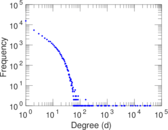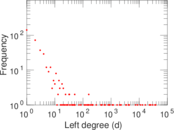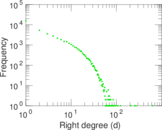### Cumulative degree distribution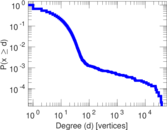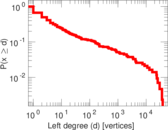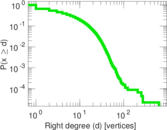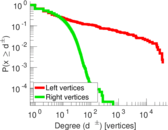### Lorenz curve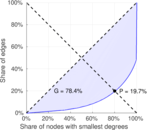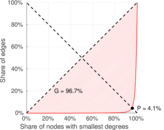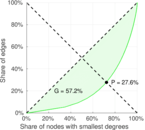### Spectral distribution of the adjacency matrix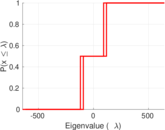### Spectral distribution of the normalized adjacency matrix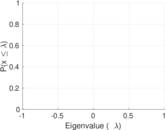### Spectral distribution of the Laplacian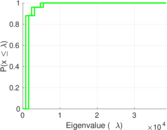### Spectral graph drawing based on the adjacency matrix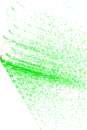### Spectral graph drawing based on the Laplacian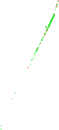### Spectral graph drawing based on the normalized adjacency matrix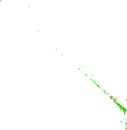### Degree assortativity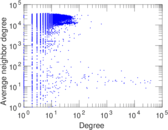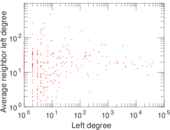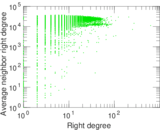### Zipf plot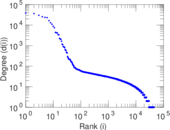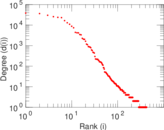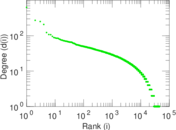### Hop distribution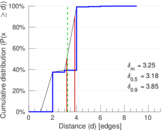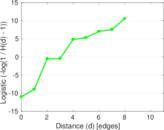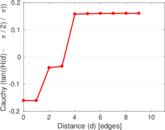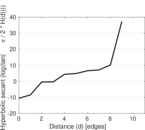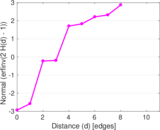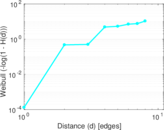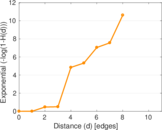### Double Laplacian graph drawing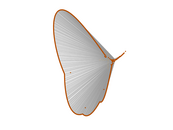### Delaunay graph drawing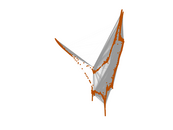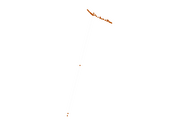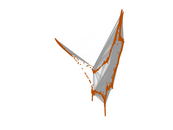### Edge weight/multiplicity distribution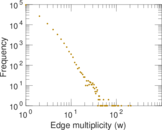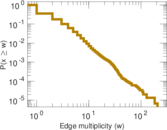### Temporal distribution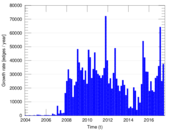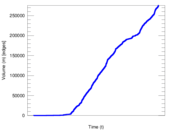### Temporal hop distribution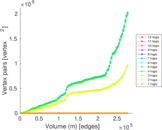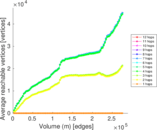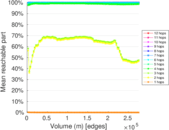### Diameter/density evolution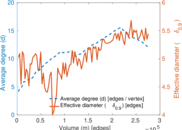### Matrix decompositions plots